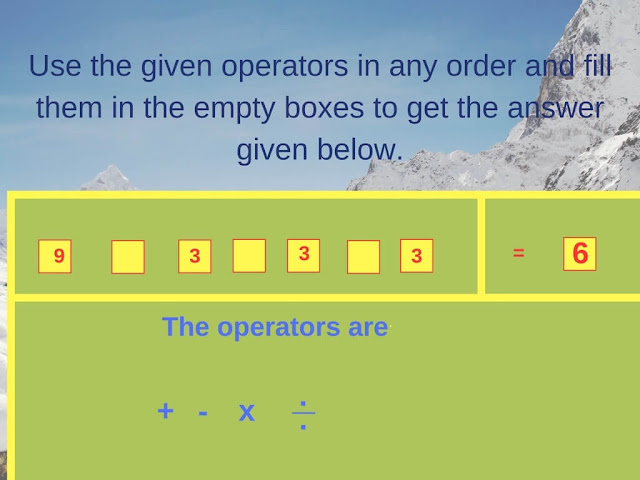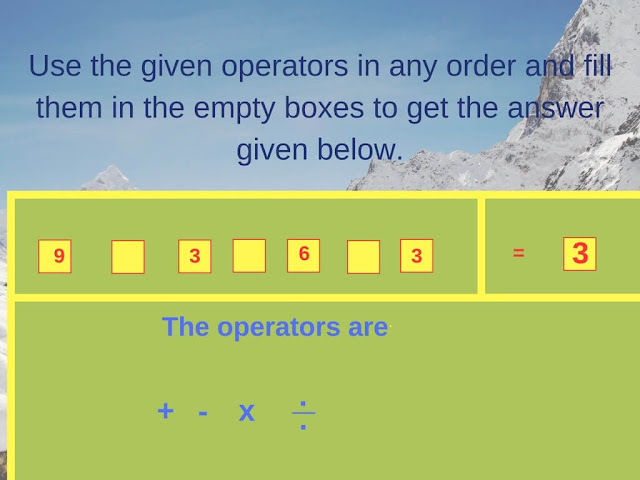In these some equation is given. However, for this equation, only numbers are given. One side of these numbers is the equation answers and another side there are numbers on which one has to apply the given operators to get the right side of the equation. These operators can be used as many times as one wants and standard BODMAS rules apply while solving these equations.

These are easy mathematical equations. Try to solve these equations without looking at the answers. Answers to these math brainteasers will be provided later. However, do post your answers for these puzzles in the comment section of this post.1. Can you make this Maths Equation Correct?2. Can you solve this Maths Equation Brain Teaser?3. Can you correct this Maths Equation?4. Can you correct these Maths Equations?5. Can you solve these Maths Equations puzzles?

Please check out Maths and Fun Brain Teasers as listed below

## List of Maths Puzzles and Brain Teasers

1. Fun Maths Number Brain Teasers for Kids with Answers: These are the Easy Fun Maths Number Brain Teasers for Kids in which one has to find the missing number in the square.

2. Very Tricky Brain-Cracking Questions for Adults: These are very tough brain-cracking Maths and Logical Questions for adults.

3. Easy Maths Picture Puzzles with answers for kids: These are Picture Maths Puzzles for kids in which your challenge is to find the missing number in the Mathematical patterns.

4. Easy Mathematical Brain Teasers with Answers For Kids: These are easy and Fun Maths Brain Teasers that will challenge your mind.